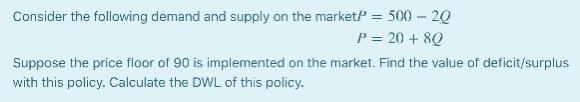### Create an Account

Already have account?

### Forgot Your Password ?

Home / Questions / Flag Consider the following demand and supply on the marketP=500−2QP=20+8Q Suppose the pr...

# Flag Consider the following demand and supply on the marketP=500−2QP=20+8Q Suppose the price floor of 90 is implemented on the market. Find the value of deficit/surplus with this policy. Calculate

Consider the following demand and supply on the marketP=500−2QP=20+8Q

Suppose the price floor of 90 is implemented on the market. Find the value of deficit/surplus with this policy. Calculate the DWL of this policy.Apr 19 2021 View more View Less

#### Answer (Solved)Subscribe To Get Solution